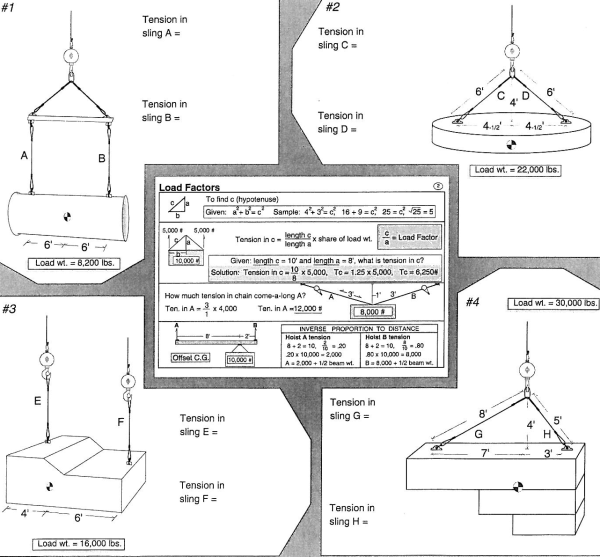Filter by Category
Filter by Category

# Rigging Training Workshop: Center of Gravity & Load TensionMany things affect the distribution of weight being lifted at various pick points on a load:

1. Where the slings or lifting attachments are secured in relationship to the load’s CG.
2. If the pick points are not equidistant from the CG, then the weight distribution will probably be unequal.
3. If the slings lifting the load are not in a straight vertical plane, there will be added tension in the slings due to the angle.

Using Panel 2 of ITI’s Journeyman Rigger’s Reference Card, find the solutions to the problems below. Focus on identifying how the weight of each load is distributed in regard to the respective pick points. If an angle is involved, how much additional load is introduced to the sing(s)?

For the answer to this workshop scroll below.

Happy Trails to all of my Crane and Rigging Friends,

Mike Parnell

P.S.  Center of Gravity & Sling Tensions are demonstrated in our Intermediate Rigging and Master Rigger  Courses.

This is an article from The Professional Rigger newsletter, 1991.

CG & Sling Tensions Workshop Solutions:

#1

To find tension in A
6+6=12, 6/12=.5  .5x8, 200=4,100 lbs
(minimum wire rope diameter = ½”)

To find tension in B
6+6=12, 6/12=.5  .5x8, 200=4,100 lbs
(minimum wire rope diameter = ½”)

#2

To find tension in C
6/4=1.5, 1.5x(22,00/2)=16,500 lbs
(minimum wire rope diameter = 1”)

To find tension in D
6/4=1.5, 1.5x(22,00/2)=16,500 lbs
(minimum wire rope diameter = 1”)

#3

To find tension in E
4+6=10, 6/10=.6  .6x16,000=9,600 lbs
(minimum wire rope diameter = ¾”)

To find tension in F
4+6=10, 4/10=.4  .4x16,000=6,400 lbs
(minimum wire rope diameter = 5/8”)

#4

To find tension in G
7+3=10, 3/10=.3  .3x30,000=9,000  9,000x(8/4)=18,000 lbs
(Minimum wire rope diameter = 1 1/8”)

To find tension in H
7+3=10, 7/10=.7  .7x30,000=21,000 21,000x(5/4)=26,250 lbs
(minimum wire rope diameter = 1 3/8”)